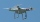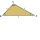# Reason + triangle - math problems

#### Number of problems found: 57

• Wheel gearA drive wheel of radius 2 is connected to a drive wheel of radius 1 by a pulley of length 17. What is the distance between the wheel axles?
• Divide an isosceles triangleHow to divide an isosceles triangle into two parts with equal contents perpendicular to the axis of symmetry (into a trapezoid and a triangle)?
• Metal ballsFour metal balls with a diameter of 5 cm are placed in a measuring cylinder with an inner diameter of 10 cm. What is the smallest water volume to be poured into the cylinder so that all balls are below the water level?The nine-meter poplar casts a shadow 16.2 m long. How long does a shadow cast by Peter at the same time, if it is 1.4 m high?
• Integer sidesA right triangle with an integer length of two sides has one leg √11 long. How much is its longest side?
• DodecagonCalculate the size of the smaller of the angles determined by lines A1 A4 and A2 A10 in the regular dodecagon A1A2A3. .. A12. Express the result in degrees.
• ProbabilityHow probable is a randomly selected three-digit number divisible by five or seven?
• AnnulusTwo concentric circles with radii 1 and 9 surround the annular circle. This ring is inscribed with n circles that do not overlap. Determine the highest possible value of n.
• A droneA flying drone aimed the area for an architect. He took off perpendicularly from point C to point D. He was at the height of 300 m above ABC's plane. The drone from point D pointed at a BDC angle of 43°. Calculate the distance between points C and B in me
• Mysterious areaThe trapezoid ABCD is given. Calculate its area if the area of the DBC triangle is 27 cm2.
• Three parallelsThe vertices of an equilateral triangle lie on 3 different parallel lines. The middle line is 5 m and 3 m distant from the end lines. Calculate the height of this triangle.
• Land boundaryThe land has the shape of a right triangle. The hypotenuse has a length of 30m. The circumference of the land is 72 meters. What is the length of the remaining sides of the land boundary?
• Circular railwayThe railway connects in a circular arc the points A, B, and C, whose distances are | AB | = 30 km, AC = 95 km, BC | = 70 km. How long will the track from A to C?
• Coordinates of square verticesI have coordinates of square vertices A / -3; 1/and B/1; 4 /. Find coordinates of vertices C and D, C and D. Thanks, Peter.
• Depth anglesAt the top of the mountain stands a castle, which has a tower 30 meters high. We see the crossroad in the valley from the top of the tower and heel at depth angles of 32° 50 'and 30° 10'. How high is the top of the mountain above the crossroad
• Tangent spheresA sphere with a radius of 1 m is placed in the corner of the room. What is the largest sphere size that fits into the corner behind it? Additional info: Two spheres are placed in a corner of a room. The spheres are each tangent to the walls and floor and
• Quarter circleWhat is the radius of a circle inscribed in the quarter circle with a radius of 100 cm?
• Equilateral triangle ABCIn the equilateral triangle ABC, K is the center of the AB side, the L point lies on one-third of the BC side near the point C, and the point M lies in the one-third of the side of the AC side closer to the point A. Find what part of the ABC triangle cont
• ProveProve that k1 and k2 are the equations of two circles. Find the equation of the line that passes through the centers of these circles. k1: x2+y2+2x+4y+1=0 k2: x2+y2-8x+6y+9=0
• TrianglesFive sticks with a length of 2,3,4,5,6 cm. How many ways can you choose three sticks to form three sides of a triangle?

Do you have an exciting math question or word problem that you can't solve? Ask a question or post a math problem, and we can try to solve it.

We will send a solution to your e-mail address. Solved examples are also published here. Please enter the e-mail correctly and check whether you don't have a full mailbox.

See also our trigonometric triangle calculator. Reason - math problems. Triangle Problems.